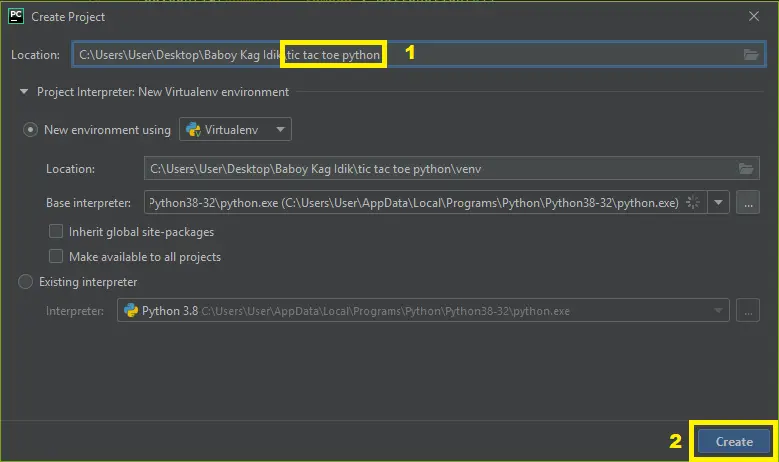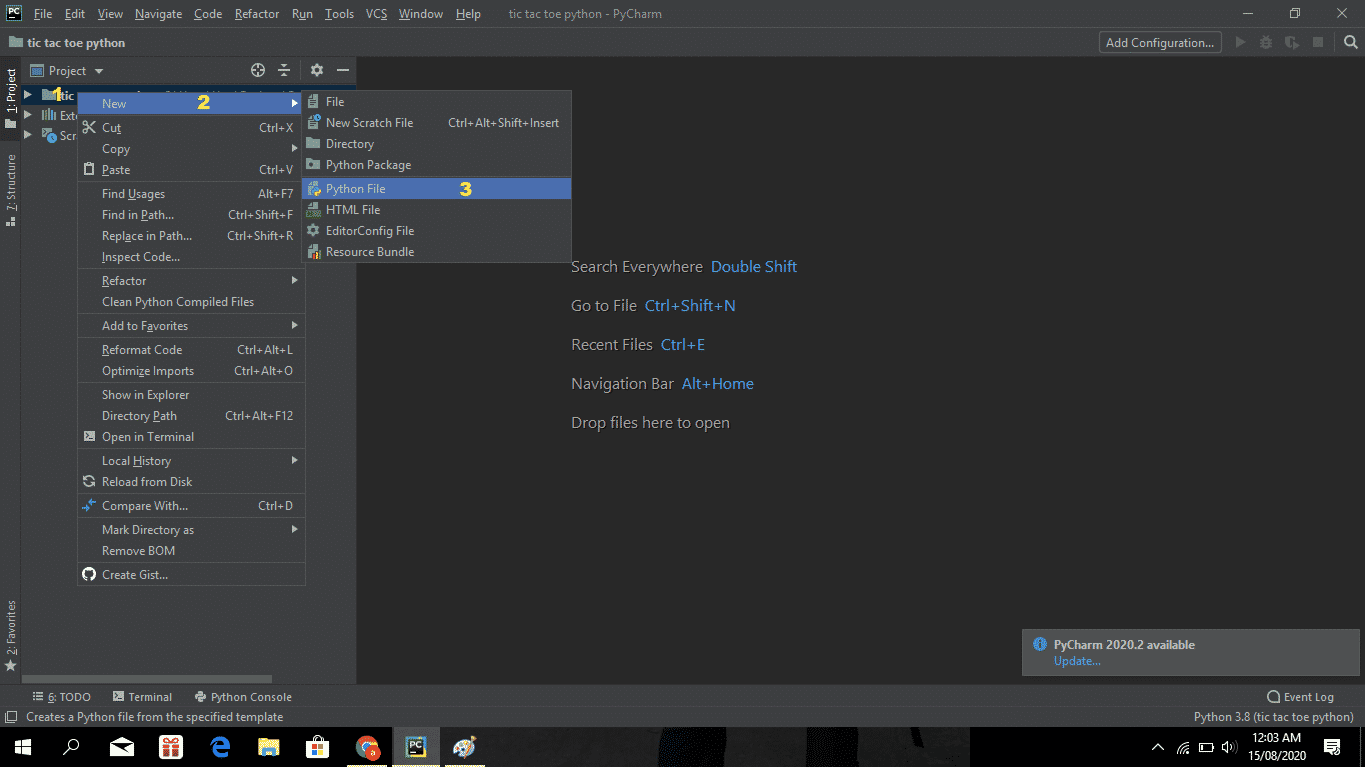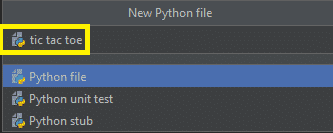# Tic Tac Toe In Python With Source Code

The Tic Tac Toe In Python is written in Python programming language, this Tic Tac Toe Game In Python is a simple GUI based strategy game board that is very easy to understand and use.

All the playing rules are the same just like we play in real-time tic-tac-toe, it is a simple multiplayer game.

## Tic Tac Toe Game In Python: Project Information

A Tic Tac Toe In Python Using Tkinter game starts with a GUI board. First, the player has to click on any box to start the game. It contains the Minimax algorithm; which is a decision rule used for a two-player game.

A simple Tic Tac Toe 2 Player 2D GUI is provided for easy gameplay. The gameplay design is so simple that users won’t find it difficult to use and understand.

Anyway, if you want to level up your knowledge in programming especially games in Python, try this new article I’ve made for you Code For Game in Python: Python Game Projects With Source Code.

To start creating a Tic Tac Toe Game In Python, make sure that you have PyCharm IDE installed on your computer.

By the way, if you are new to Python programming and don’t know what Python IDE is and its usage, I have here a list of the Best Python IDE for Windows, Linux, and Mac OS that will suit you.

## How to Create Tic Tac Toe in Python with Source Code

Time needed: 5 minutes

These are the Steps on How to Create a Tic Tac Toe Game In Python With Source Code

• Step 1: Create a project name.

First, open Pycharm IDE and then create a “project name” After creating a project name click the “create” button.• Step 2: Create a python file.

Second, after creating a project name, “right click” your project name and then click “new” After that click the “python file“.• Step 3: Name your python file.

Third, after creating a Python file, Name your Python file after that click “enter“.• Step 4: The actual code.

You are free to copy the code given below and download the full source code below.

## Code Explanations

### 1. The Code Given Below Is For Importing Modules

Code:

``````from tkinter import Tk,ttk,Button
from tkinter import messagebox
from random import randint``````

Explanation:

The code is given which is importing all modules or libraries.

### 2. The Code Given Below Is For The Module Lay Out

Code:

``````def SetLayout(id,player_symbol):
if id==1:
b1.config(text= player_symbol)
b1.state(['disabled'])
elif id==2:
b2.config(text= player_symbol)
b2.state(['disabled'])
elif id==3:
b3.config(text= player_symbol)
b3.state(['disabled'])
elif id==4:
b4.config(text= player_symbol)
b4.state(['disabled'])
elif id==5:
b5.config(text= player_symbol)
b5.state(['disabled'])
elif id==6:
b6.config(text= player_symbol)
b6.state(['disabled'])
elif id==7:
b7.config(text= player_symbol)
b7.state(['disabled'])
elif id==8:
b8.config(text= player_symbol)
b8.state(['disabled'])
elif id==9:
b9.config(text= player_symbol)
b9.state(['disabled'])``````

Explanation:

In this module which is setting the layout of its player symbol.

### 3. The Code Given Below Is For The Check Winner Module

Code:

``````def CheckWinner():
global mov
winner = -1

if(1 in p1) and (2 in p1) and (3 in p1):
winner = 1
if(1 in p2) and (2 in p2) and (3 in p2):
winner = 2

if(4 in p1) and (5 in p1) and (6 in p1):
winner = 1
if(4 in p2) and (5 in p2) and (6 in p2):
winner = 2

if(7 in p1) and (8 in p1) and (9 in p1):
winner = 1
if(7 in p2) and (8 in p2) and (9 in p2):
winner = 2

if(1 in p1) and (4 in p1) and (7 in p1):
winner = 1
if(1 in p2) and (4 in p2) and (7 in p2):
winner = 2

if(2 in p1) and (5 in p1) and (8 in p1):
winner = 1
if(2 in p2) and (5 in p2) and (8 in p2):
winner = 2

if(3 in p1) and (6 in p1) and ( 9 in p1):
winner = 1
if(3 in p2) and (6 in p2) and (9 in p2):
winner = 2

if(1 in p1) and (5 in p1) and ( 9 in p1):
winner = 1
if(1 in p2) and (5 in p2) and (9 in p2):
winner = 2

if(3 in p1) and (5 in p1) and ( 7 in p1):
winner = 1
if(3 in p2) and (5 in p2) and (7 in p2):
winner = 2

if winner ==1:
messagebox.showinfo(title="Congratulations.",
message="Player 1 is the winner")
elif winner ==2:
messagebox.showinfo(title="Congratulations.",
message="Player 2 is the winner")
elif mov ==9:
messagebox.showinfo(title="Draw",
message="It's a Draw!!")``````

Explanation:

In this module which is checking the winner of two players.

### 4. The Code Given Below Is For The Module Button Click

Code:

``````def ButtonClick(id):
global ActivePlayer
global p1,p2
global mov

if(ActivePlayer ==1):
SetLayout(id,"X")
p1.append(id)
mov +=1
root.title("Tic Tac Toe : Player 2")
ActivePlayer =2

elif(ActivePlayer==2):
SetLayout(id,"O")
p2.append(id)
mov +=1
root.title("Tic Tac Toe : Player 1")
ActivePlayer =1
CheckWinner()``````

Explanation:

In this module which is the button click of two players and its conditions.

### 5. The Code Given Below Is For The Module Restart

Code:

``````def Restart():
global p1,p2,mov,ActivePlayer
p1.clear(); p2.clear()
mov,ActivePlayer = 0,1
root.title("Tic Tac Toe : Player 1")
EnableAll()``````

Explanation:

In this module which is the restarting or the new game module of the game.

## Complete Source Code

``````from tkinter import Tk,ttk,Button
from tkinter import messagebox
from random import randint

ActivePlayer = 1
p1 = []
p2 = []
mov = 0

def SetLayout(id,player_symbol):
if id==1:
b1.config(text= player_symbol)
b1.state(['disabled'])
elif id==2:
b2.config(text= player_symbol)
b2.state(['disabled'])
elif id==3:
b3.config(text= player_symbol)
b3.state(['disabled'])
elif id==4:
b4.config(text= player_symbol)
b4.state(['disabled'])
elif id==5:
b5.config(text= player_symbol)
b5.state(['disabled'])
elif id==6:
b6.config(text= player_symbol)
b6.state(['disabled'])
elif id==7:
b7.config(text= player_symbol)
b7.state(['disabled'])
elif id==8:
b8.config(text= player_symbol)
b8.state(['disabled'])
elif id==9:
b9.config(text= player_symbol)
b9.state(['disabled'])

def CheckWinner():
global mov
winner = -1

if(1 in p1) and (2 in p1) and (3 in p1):
winner = 1
if(1 in p2) and (2 in p2) and (3 in p2):
winner = 2

if(4 in p1) and (5 in p1) and (6 in p1):
winner = 1
if(4 in p2) and (5 in p2) and (6 in p2):
winner = 2

if(7 in p1) and (8 in p1) and (9 in p1):
winner = 1
if(7 in p2) and (8 in p2) and (9 in p2):
winner = 2

if(1 in p1) and (4 in p1) and (7 in p1):
winner = 1
if(1 in p2) and (4 in p2) and (7 in p2):
winner = 2

if(2 in p1) and (5 in p1) and (8 in p1):
winner = 1
if(2 in p2) and (5 in p2) and (8 in p2):
winner = 2

if(3 in p1) and (6 in p1) and ( 9 in p1):
winner = 1
if(3 in p2) and (6 in p2) and (9 in p2):
winner = 2

if(1 in p1) and (5 in p1) and ( 9 in p1):
winner = 1
if(1 in p2) and (5 in p2) and (9 in p2):
winner = 2

if(3 in p1) and (5 in p1) and ( 7 in p1):
winner = 1
if(3 in p2) and (5 in p2) and (7 in p2):
winner = 2

if winner ==1:
messagebox.showinfo(title="Congratulations.",
message="Player 1 is the winner")
elif winner ==2:
messagebox.showinfo(title="Congratulations.",
message="Player 2 is the winner")
elif mov ==9:
messagebox.showinfo(title="Draw",
message="It's a Draw!!")

def ButtonClick(id):
global ActivePlayer
global p1,p2
global mov

if(ActivePlayer ==1):
SetLayout(id,"X")
p1.append(id)
mov +=1
root.title("Tic Tac Toe : Player 2")
ActivePlayer =2

elif(ActivePlayer==2):
SetLayout(id,"O")
p2.append(id)
mov +=1
root.title("Tic Tac Toe : Player 1")
ActivePlayer =1
CheckWinner()

def AutoPlay():
global p1; global p2
Empty = []
for cell in range(9):
if(not((cell +1 in p1) or (cell +1 in p2))):
Empty.append(cell+1)
try:
RandIndex = randint(0,len(Empty) -1)
ButtonClick(Empty[RandIndex])
except:
pass

def EnableAll():
b1.config(text= " ")
b1.state(['!disabled'])
b2.config(text= " ")
b2.state(['!disabled'])
b3.config(text= " ")
b3.state(['!disabled'])
b4.config(text= " ")
b4.state(['!disabled'])
b5.config(text= " ")
b5.state(['!disabled'])
b6.config(text= " ")
b6.state(['!disabled'])
b7.config(text= " ")
b7.state(['!disabled'])
b8.config(text= " ")
b8.state(['!disabled'])
b9.config(text= " ")
b9.state(['!disabled'])

def Restart():
global p1,p2,mov,ActivePlayer
p1.clear(); p2.clear()
mov,ActivePlayer = 0,1
root.title("Tic Tac Toe : Player 1")
EnableAll()

root = Tk()
root.title("Tic Tac toe : Player 1")
st = ttk.Style()
st.configure("my.TButton", font=('Chiller',24,'bold'))

b1 = ttk.Button(root, text=" ", style="my.TButton")
b1.config(command = lambda : ButtonClick(1))

b2 = ttk.Button(root, text=" ",style ="my.TButton")
b2.config(command = lambda : ButtonClick(2))

b3= ttk.Button(root, text=" ",style="my.TButton")
b3.config(command = lambda : ButtonClick(3))

b4 = ttk.Button(root, text=" ",style="my.TButton")
b4.config(command = lambda : ButtonClick(4))

b5 = ttk.Button(root, text=" ",style="my.TButton")
b5.config(command = lambda : ButtonClick(5))

b6 = ttk.Button(root, text=" ",style="my.TButton")
b6.config(command = lambda : ButtonClick(6))

b7 = ttk.Button(root, text=" ",style="my.TButton")
b7.config(command = lambda : ButtonClick(7))

b8 = ttk.Button(root, text=" ",style="my.TButton")
b8.config(command = lambda : ButtonClick(8))

b9 = ttk.Button(root, text=" ",style="my.TButton")
b9.config(command = lambda : ButtonClick(9))

Button(text="New Game..", font=('Courier new', 22, 'bold'), bg='blue', fg='white',
border=5, width=4,command = lambda :Restart()).grid(row=0, column=1, sticky="we")
root.resizable(0,0)
root.mainloop()
``````

## Summary

The Tic Tac Toe Game In Python is written in Python programming language, Python is very easy to research the syntax emphasizes readability and it is able to reduce time ingesting in developing.

This is a simple GUI-based system, specially written for beginners. The Tic Tac Toe project with source code is free to download. Use for education purposes only!

Also, this tutorial is the simplest way for beginners or students to enhance their logical skills in programming. This game project is a way for students or beginners to design and develop games.